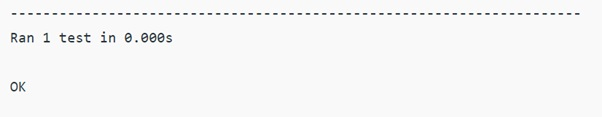# Best way to assert for numpy.array() equality?

Learn about the assert method for numpy.array() equality in Python?
Submitted by Pranit Sharma, on December 28, 2022

NumPy is an abbreviated form of Numerical Python. It is used for different types of scientific operations in python. Numpy is a vast library in python which is used for almost every kind of scientific or mathematical operation. It is itself an array which is a collection of various methods and functions for processing the arrays.

## Assert for numpy.array() equality

Suppose we are given two numpy arrays and we need to compare them. There is a method called array._eq_() but it returns a new array. We need to find the best way to assert for equality.

For this purpose, using self.assertEqual(arr1.tolist(), arr2.tolist()) is the easiest way of comparing arrays.

We will get all the error descriptions. Although it will not work well with np.nan, since np.nan != np.nan and the self.assertEqual attempt won't be able to account for that.

Let us understand with the help of an example,

## Python code to demonstrate the best way to assert for numpy.array() equality

```# Import numpy
import numpy as np

# Import unittest
import unittest

# Encapsulating everything in a class
class compare_arrays(unittest.TestCase):

# Defining a function
def fun(self):
# Creating two numpy arrays
arr = np.array([1,2,3,4,5,6,7,8,9,10])
arr2 = np.array([1,2,3,4,5,6,7,8,9,10])

# Display original arrays
print("Original array 1:\n",arr,"\n")
print("Original array 2:\n",arr2,"\n")

# Compare two arrays
self.assertEqual(arr,arr2,"Both arrays are equal")

if __name__ == '__main__':
unittest.main()
```

Output: